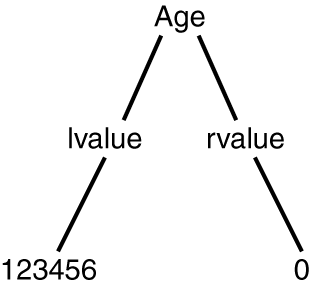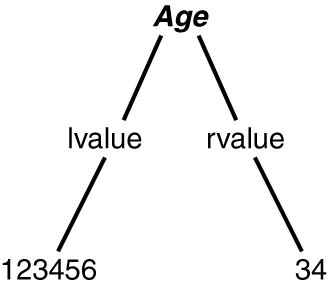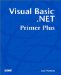# Behind the Scenes: The Visual Basic .NET Symbol Table

Although it seemed in the previous chapter that Visual Basic .NET instantaneously created the new variable Age for use in your program, a lot of things were going on between the time you pressed the Enter key and when the cursor moved to the next line. Let's look under the hood to see what Visual Basic .NET did in that brief moment of time.

First, Visual Basic .NET parsed the line you typed to see if it obeyed all the syntax rules. If you had misspelled Dim as Dem , or misspelled Integer as Intger , or made some other spelling mistake in a keyword, Visual Basic .NET would have told you so by sending a syntax error message. If you had violated one of the naming rules for Age , Visual Basic .NET would have issued a syntax error message to you. Because you spelled everything correctly, placed each keyword in the proper place, and followed the proper naming rules for the variable, Visual Basic .NET was happy.

#### The Symbol Table

Thus far, Visual Basic .NET knows that the Dim statement is properly written. The next thing Visual Basic .NET does is make sure it can create a variable name Age with the Integer properties you gave it. To do this, it first examines something called a symbol table. A symbol table is a table that Visual Basic .NET creates automatically in the background to keep track of each and every data item used in a program. Visual Basic .NET uses the symbol table to track dozens of attributes about each data item, and two of the primary attributes kept in the symbol table are the name of the variable and its data type.

After Visual Basic .NET is happy that you obeyed its syntax rules, it scans its symbol table to see if you have already dimensioned (that is, defined) a variable with the name Age . If you have already created a variable named Age in the program, Visual Basic .NET displays a squiggly line under the word Age in the Dim statement. If you then try to compile the program, Visual Basic .NET would issue an error message like this:

` Local variable Age is already declared in the current block. `

This message tells you that you tried to create a variable that has the same name as a variable that already exists. If you created two variables with the same name, Visual Basic .NET wouldn't know which one to use; therefore, it issues an error message to prevent such confusion.

If there is not another Age variable already defined in the program, Visual Basic .NET attempts to find a place in memory to store the Age variable.

Actually, Visual Basic .NET cannot store something by itself; it needs the permission of the operating system. For Visual Basic .NET, the operating system is Microsoft Windows. Therefore, Visual Basic .NET sends a message to Windows saying, "Hey, Windows! My programmer is trying to create an Integer variable. Can you give me a memory address that currently is not being used where I can store 4 bytes of data?" (Table 4.1 tells you that an Integer data type needs 4 bytes of memory, remember?)

The Windows memory manager then looks through its available memory blocks for 4 bytes of unused memory that it can release for use by Visual Basic .NET. If the memory manager finds 4 bytes of free memory (which it usually can), it sends a return message that says: "Hey, Visual Basic .NET! You can use the 4 bytes of memory starting at memory address 123456." (Obviously, the actual memory address will vary. Also, the memory address is expressed using the hexadecimal numbering system described in Chapter 2. I use the decimal numbering system here just to keep things simple.) Visual Basic .NET then stores that memory address (123456) in its symbol table, along with the variable's name ( Age ) and its data type ( Integer ). If the memory manager cannot not find 4 bytes of free memory, it tells Visual Basic .NET it is out of memory, and Visual Basic .NET passes the bad news along to you in the form of an error message. If all goes well, Visual Basic .NET creates a variable named Age for use in your program.

NoteIt is pretty rare for an "out of memory" error message to occur at the time you are writing a program (that is, design time). You are more likely to see such a message after the program starts running. You'll learn more about "out of memory" error messages and how to fix them in later chapters.

#### lvalue and rvalue

Figure 4.1 shows what is associated with the Age variable in the symbol table.

##### Figure 4.1. The lvalue and rvalue for Age .As you can see in Figure 4.1, the left value in the diagram, called the lvalue , represents the memory address where the variable named Age is stored. The right value in the diagram, rvalue , is what is stored at that memory address. You can think of the lvalue as the "where" and the rvalue as the "what."

Because Visual Basic .NET initializes all newly created variables with the value , the rvalue for Age is . In other words, the lvalue is where Age is located in the computer's memory, and the rvalue is what is actually stored at that memory location. Think of a variable as a bucket that can hold a data item; the lvalue is where the bucket is located, and the rvalue is what's inside the bucket. The variable's type specifier tells how big the bucket is. Visual Basic .NET uses the lvalue to locate the variable named Age any time you need to use it in your program.

The Visual Basic .NET symbol table for your program might look as shown in Table 4.3.

##### Table 4.3. A Visual Basic .NET Symbol Table

Variable Name

Data Type

Bytes

Lvalue

Other Attributes

Age

Integer

4

123456

The column names in Table 4.3 should be familiar to you now. The last column, Other Attributes, indicates that the symbol table is considerably more complex than the one we are showing here. It would not be unusual to have several dozen attributes maintained in a symbol table for each data item. Table 4.3 just shows the attributes that we are currently interested in.

#### Operands and Operators

Now that you have a variable named Age available for use in your program, you should do something with it. Consider the following Visual Basic .NET program statement:

` Age = 34 `

This is a valid Visual Basic .NET program statement that assigns the numeric value 34 to the Age variable. This program statement uses the assignment operator, the equal sign ( = ), to change the value of Age from its current value of to 34 . To Visual Basic .NET, a valid assignment statement is expressed as follows :

Operand1

AssignmentOperator

Operand2

Age

=

`  34  `

In Visual Basic .NET, a properly constructed assignment statement has these three parts :

• Operand1 Often a variable name

• AssignmentOperator The equal sign

• Operand2 The value to be assigned into Operand1

Because the assignment operator requires two operands, it is called a binary operator. If either operand is missing, or if the operator is missing, Visual Basic .NET issues an error message.

#### How Visual Basic .NET Processes an Assignment Statement

When Visual Basic .NET sees this program statement:

` Age = 34 `

it breaks the statement into three parts: Operand1 , AssignmentOperator , and Operand2 . When Visual Basic .NET reads the equal sign, it knows there must be two operands. Because this program statement does have two operands, Visual Basic .NET proceeds with the next phase of the program statement.

In the next phase, Visual Basic .NET goes to the symbol table to see if Operand1 has been defined. Visual Basic .NET scans through the symbol table, looking for Operand1 (that is, Age ). If you have properly defined the variable named Age , Visual Basic .NET finds an entry for Operand1 , much like the entry shown in Figure 4.2. Visual Basic .NET then looks at the other attributes in the symbol table for Operand1 and does the following sequence of operations:

1. It looks up the lvalue of Operand1 (that is, 123456 in Figure 4.2).

2. It formats the value in Operand2 (that is, 34 ) into an Integer data type, using the required number of bytes for the data type (that is, 4 bytes for an Integer variable).

3. It moves those 4 bytes of data into memory, starting at the memory address specified by the variable's lvalue .

##### Figure 4.2. The lvalue and rvalue for Age after the assignment statement is processed .When Visual Basic .NET finishes these three steps, the information previously shown in Figure 4.1 looks like the information shown in Figure 4.2.

As you can see, Visual Basic .NET does a lot simply to change the value of Age from to 34 .Visual Basic .NET Primer Plus
ISBN: 0672324857
EAN: 2147483647
Year: 2003
Pages: 238
Authors: Jack Purdum

Similar book on Amazon